###Mechanization

When most of us begin the learn abacus we are taught to add and subtract complementary numbers. This is a big part of the process of mechanization. Occasionally the process of mechanization can be looked at in a slightly different way. Specifically when adding or subtracting numbers like 5, 6, 7 & 8  there is an alternative. Here is a pattern that works very well for some bead configurations.

By way of explanation, I'll offer some simple examples.

Example 1:  5 + 8 = 13

Rod C is the unit rod. Set 5 on rod C.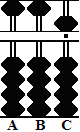Conventionally we're taught to subtract the compliment of 8 (in this case 2) from the unit rod. This leaves 3. From here we continue by adding a 10 bead to make 13. This is completely correct but as with all things abacus there may be another way to think about it.

When adding 8 to 5 on rod C all we really need to do is move the beads up 5 and up 3; in essence up 8. Then add the 10 bead to equal 13.

In other words; in order to add 8 all we do is up 8.

Thinking this way the problem 5 + 8 = 13 becomes: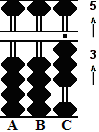up 5 and up 3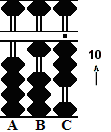then up 10 to equal 13

Example 2:  7 + 7 = 14

Rod C is the unit rod. Set 7 on rod C.In this example, when we add 7 to 7 we're taught to subtract 3 which is the complement of 7. This leaves 4. Then add a 10 bead to equal 14. Once again completely correct. But is there an alternative?

In order to add 7 to 7 all we have to do is move up 5 and up 2; in essence up 7. Then add the 10 bead to rod B to equal 14.

In other words; in order to add 7 all we do is up 7.

Thinking this way the problem 7 + 7 = 14 becomes: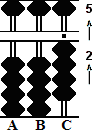up 5 and up 2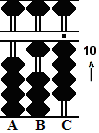then up 10 to equal 14

Other instances where this thought process can be used are:
5+6,  5+7,  5+9,  6+6,  6+7,  6+8, 7+6, 8+6

SUBTRACTION:

In subtraction the process works the same way but in reverse.

Example 3:  14 - 8 = 6

Rod C is the unit rod. Set 14 on your soroban.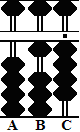First subtract the 10 bead. Then we're taught to add the compliment of 8 (which is 2). This leaves 6. While perfectly valid here's an alternative.

First subtract the 10 bead. Then on the unit rod move down 5 and down 3.

In other words; in order to subtract 8 all we do is down 8.

Thinking this way the problem 14 - 8 = 6 becomes:down 10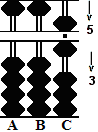then down 5 and down 3 to equal 6.

Example 4:  13 - 6 = 7

Rod C is the unit rod. Set 13 on your soroban.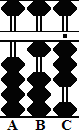First subtract the 10 bead. Then we're taught to add the compliment of 6 (which is 4). This leaves 7. Try it this way. First subtract the 10 bead. Then on the unit rod move down 5 and down 1.

In other words; in order to subtract 6 all we do is down 6.

13 - 6 = 7 becomesdown 10then down 5 and down 1 to equal 7.

Other instances where this thought process can be used are:
11-6,  12-6,  12-7,  13-7,  13-8,  14-6, 14-7, 14-9

It's evident that no matter the thought process, the bead movements in the two schools of thinking are exactly same. Each is equally efficient. One of the most fascinating things about learning soroban is that everyone will come to this in their own way.

 For those students who are new to numbers

Back to Abacus: Mystery of the Bead

Print Page (.pdf format,97kb)